# 跟Frame长像稍不同的LabelFrame组件讲解

```from tkinter import *

root=Tk()

lfr1=LabelFrame(root,text='  选择喜欢的水果  ') # 创建LabelFrame组件

var=IntVar(value=1)

root.mainloop()```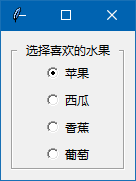这就是我们常看到的LabelFrame组件在窗体的外貌，一个Frame组件加一个标签，如果你在创建LabelFrame的代码里，去除 text='选择喜欢的水果'，那外表看上去就是一个Frame组件，LableFrame组件也有relief参数，也可以改变样式，如：

```from tkinter import *

root=Tk()

lfr1=LabelFrame(root,text='  选择喜欢的水果  ',relief='raised') # 创建LabelFrame组件

print(lfr1.keys())   # 输出组件的相关参数项

var=IntVar(value=1)

root.mainloop()```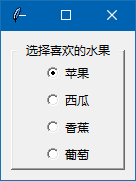LableFrame组件上的标签，可以改变位置到组件上的其它位置上，要就要用到 labelanchor参数，这个参数支持标签处于 'e''s''w''n''es''ws''en''wn''ne''nw''se''sw'  12个位置。我们用代码为测试一下：

```from tkinter import *

root=Tk()

lfr1=LabelFrame(root,text='选\n择\n喜\n欢\n的\n水\n果',labelanchor='w')

var=IntVar(value=1)

root.mainloop()```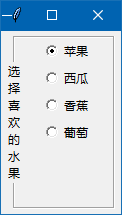如果你只是用LableFrame的基本功能，我看只要掌握以上这几个参数也差不多了。但LableFrame还有一些参数，你其实也可以了解一下。我们在创建LableFrame组件后的代码，再加入 print(lfr1.keys()) 代码，在运行后，在命令行里会输出相关的参数：

['bd', 'borderwidth', 'class', 'fg', 'font', 'foreground', 'labelanchor', 'labelwidget', 'relief', 'text', 'background', 'bg', 'colormap', 'container', 'cursor', 'height', 'highlightbackground', 'highlightcolor', 'highlightthickness', 'padx', 'pady', 'takefocus', 'visual', 'width']

labelwidget

```from tkinter import *

root=Tk()

img1=PhotoImage(file='16-1.png') # 创建图像对象,要自己准备好图片
la1=Label(root,text='选择喜欢的水果',image=img1,compound='left')

lfr1=LabelFrame(root,labelwidget=la1) # la1代替组件的标签

var=IntVar(value=1)

root.mainloop()```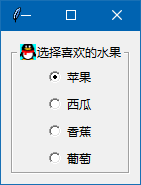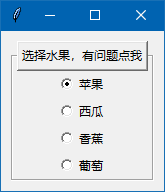```from tkinter import *
root=Tk()

lfr1=LabelFrame(root,height=2, borderwidth=1, relief='groove',labelanchor='e') # 阴凹分割线

lfr2=LabelFrame(root,height=2, borderwidth=1, relief='ridge',labelanchor='e') # 阳凸分割线

root.mainloop()```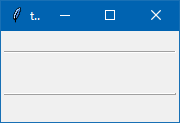<< 上一篇 下一篇 >>